## Cubics

Thursday 26 January 2006 at 9:26 pm | In Articles | 1 Comment

A problem that I give some of the students is to show that for a cubic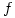that if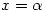and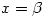are the x-coordinates of its stationary points then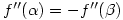. Hopefully, they will have already noticed this result in the exercises they have done.

I then ask them to show that the point of inflexion ofis at the midpoint of the turning points. This involves using the equations for the roots of a quadratic: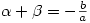and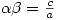but the algebra isn’t particularly nice (details are here).

It dawned on me that there’s a better way which is not only simpler to prove but gives a more powerful result: a cubic is (rotationally) symmetric about its point of inflexion.

To see this, take the cubic and translate it so that the point of inflexion is at the origin. This clearly has no effect on the symmetry so it is sufficient to prove the result in this case.

If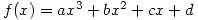has its point of inflexion at the origin then clearly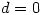and, as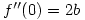, it follows that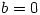so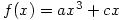. Rotate this by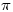about the origin, for example by applying the usual matrix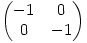, and you get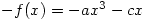which is clearly the same cubic. Hence it has rotational symmetry about the origin, and in particular, the point of inflexion at the origin is the midpoint of the stationary points.

## Some textbooks misuse infinity

Monday 18 October 2004 at 3:43 pm | In Articles | Post Comment

It’s happened again!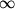used in a textbook (unnamed to protect the guilty) as if it were a real number instead of an idea. In a discussion of the formula for the acute angle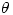between two lines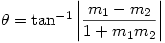the following appears:

Putting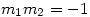gives an angle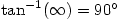, confirming the condition for the lines to be perpendicular

This is of course complete nonsense. As I’ve said before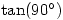doesn’t exist and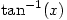is only defined on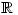ie for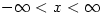The textbook was written by the examiners (which is one reason why we use it); this worries me even more.
I suppose this is better than one well-known textbook back in the eighties which solved the equation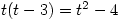by putting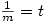then ‘showing’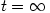or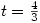. This seems to show that all linear equations are quadratics in disguise; or cubics, quartics – who knows where this nonsense leads 😕